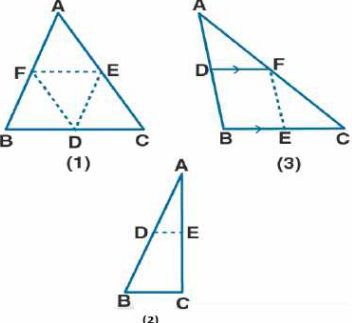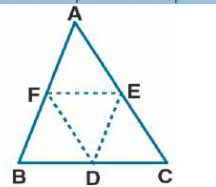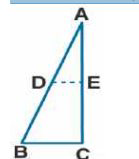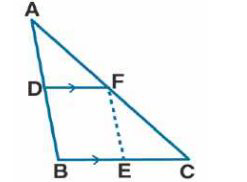# ML Aggarwal Solutions Class 9 Mathematics Solutions for Mid Point Theorem Exercise 11 in Chapter 11 - Mid Point Theorem

Question 1 Mid Point Theorem Exercise 11

(a) In the figure (1) given below, D, E, and F are mid-points of the sides BC, CA, and AB respectively of Δ

ABC. If AB = 6 cm, BC = 4.8 cm and CA = 5.6 cm, find the perimeter of (i) the trapezium FBCE (ii) the

triangle DEF.

(b) In figure (2) given below, D and E are mid-points of the sides AB and AC respectively. If

BC = 5.6 cm and ∠B = 72^{0}

(c) In figure (3) given below, D and E are mid-points of AB, BC respectively, and DF || BC. Prove that

DBEF is a parallelogram. Calculate AC if AF = 2.6 cm.(a) (i) It is given that

AB = 6 cm, BC = 4.8 cm and CA = 5.6 cm

To find: The perimeter of trapezium FBCAIt is given that

F is the mid-point of AB

We know that

BF = ½ AB = ½ × 6 = 3 cm ……. (1)

It is given that

E is the mid-point of AC

We know that

CE = ½ AC = ½ × 5.6 = 2.8 cm ……. (2)

Here F and E are the mid-point of AB and CA

FE || BC

We know that

FE = ½ BC = ½ × 4.8 = 2.4 cm …… (3)

Here

Perimeter of trapezium FBCE = BF + BC + CE + EF

Now substituting the value from all the equations

= 3 + 4.8 + 2.8 + 2.4

= 13 cm

Therefore, the perimeter of trapezium FBCE is 13 cm.

(ii) D, E and F are the midpoints of sides BC, CA and AB of Δ ABC

Here EF || BC

EF = ½ BC = ½ × 4.8 = 2.4 cm

DE = ½ AB = ½ × 6 = 3 cm

FD = ½ AC = ½ × 5.6 = 2.8 cmWe know that

Perimeter of Δ DEF = DE + EF + FD

Substituting the values

= 3 + 2.4 + 2.8

= 8.2 cm

(b) It is given that

D and E are the mid-point of sides AB and AC

BC = 5.6 cm and ∠B = 72^{0}

To find: (i) DE (ii) ∠ADEWe know that

In Δ ABC \

D and E is the mid-point of the sides AB and AC

Using mid-point theorem

DE || BC

(i) DE = ½ BC = ½ × 5.6 = 2.8 cm

(ii) ∠ADE = ∠B are corresponding angles

It is given that

∠B =

72^{0}

and BC || DE

72^{0}

(c) It is given that

D and E are the midpoints of AB and BC respectively

DF || BC and AF = 2.6 cm

To find: (i) BEF is a parallelogram

(ii) Calculate the value of AC

Proof:

(i) In Δ ABC

D is the midpoint of AB and DF || BC

F is the midpoint of AC ….. (1)

F and E are the midpoints of AC and BC

EF || AB ….. (2)

Here DF || BC

DF || BE ….. (3)

Using equation (2)

EF || AB

EF || DB ….. (4)

Using equation (3) and (4)

DBEF is a parallelogram

(ii) F is the midpoint of AC

So we get

AC = 2 × AF = 2 × 2.6 = 5.2 cm

Related Questions
Exercises

Lido

Courses

Teachers

Book a Demo with us

Syllabus

Maths
CBSE
Maths
ICSE
Science
CBSE

Science
ICSE
English
CBSE
English
ICSE
Coding

Terms & Policies

Selina Question Bank

Maths
Physics
Biology

Allied Question Bank

Chemistry
Connect with us on social media!